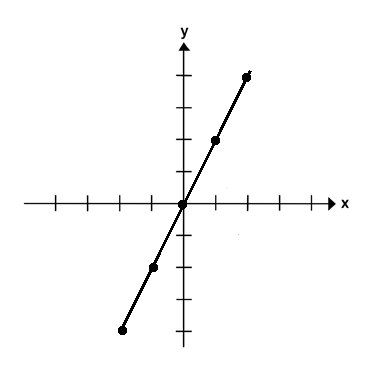Home > Grade 8 > Functions > Incorrect Linear Equations

# Incorrect Linear Equations

Directions: Based on the graph of this line, write a linear equation that is incorrect. Write an a linear equation that could be correct.### Hint

What determines the steepness of a linear equation.
What important information does slope-intercept form easily tell you about a line?

There are multiple right answers as long as the slope and y-intercepts make sense for the graph that is drawn.

Source: Sam Olderbak

## Square Root Expression

Directions: Use the digits 1 to 9, at most one time each, to fill in …

1.2.There are multiple.

3.The answer is 2

4.It could be y=2x and an incorrect equation would be y=2x -3

5.Incorrect- 0x+3
Correct-Y=2x

6.The correct equation is y=2x incorrect-y=x-2

7.Multiple as long as they match up

8.Correct y=2 incorrect y=4

9.Any positive slope could be argued (since axis scales are not necessarily known) with a y-intercept of 0. So any equation of the form y = mx, where m>0. Equations that wouldn’t work are of the form y = mx + b, where m is less than or equal to zero and/or b is not equal to 0.

10.Incorrect: y=x+2
Correct: y=2x

11.Incorrect: y= 5x-1
Correct: y=2x

•I totally agree with your statement.